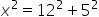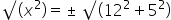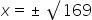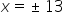Mathematics
Easy

Question

# A ladder is leaned against a tree, the height on the tree is 12 units, the ladder is 5units away from the tree, what is the length of the ladder?

## 1615313Hint:

## The correct answer is: 13

### Here we have to find that the length of ladder.Firstly , we have given that the height of ladder on tree is 12 unit and ladder away from tree is 5 unit.So, Altitude = 12 unit and Base = 5 unit.So the length of ladder = hypotenuse (Let x ) .Here we have right angled triangle , we formula for hypotenuse is:(Hypotenuse)2 = (Altitude)2 + (Base )2Taking square root on both the sides of the equation, we getSince the length of the ladder cannot be negative, x= 13 units.The correct answer is 13 unit.

In this question, we have ladder which  is leaned on tree and that formed a right angled triangle. Just use the right angled triangle property and use quadratic square root formula to find the hypotenuse.

### Related Questions to study#### With Turito Foundation.#### Get an Expert Advice From Turito.